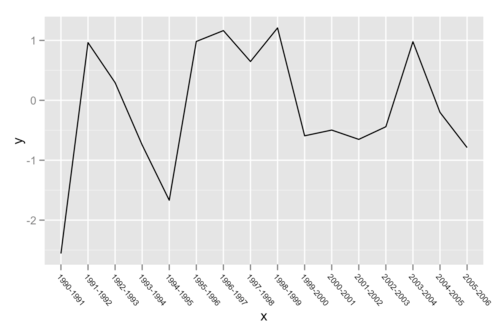Want to share your content on R-bloggers? click here if you have a blog, or here if you don't.

The syntax of ggplot2 emphasizes constructing plots by adding components, or layers, using +.

Possibly one of the most useful, but least remarked upon, consequences of this syntax is that it allows for an incredible degree of flexibility in saving and reusing components of plots. Here are two very simple examples that come up frequently for me.

I frequently make line plots where the x axis is categorical. For instance, consider the following example data:

x <- factor(paste(1990:2005,1991:2006,sep = "-"))
dat <- data.frame(x = x,y = rnorm(length(x))

which we can plot like so:

p <- ggplot(dat,aes(x = x,y = y)) + geom_line(aes(group = 1))
pObviously, we can’t keep those x axis labels like that, they’re unreadable! So I’m frequently doing something like the following:

p + opts(axis.text.x = theme_text(size = 7,
hjust = 0,
vjust = 1,
angle = 310))But who wants to type all that over and over for each plot? So instead, I just store the results of that opts() call:

x_angle <- opts(axis.text.x = theme_text(size = 7,
hjust = 0,
vjust = 1,
angle = 310))
p + x_angle

While in this case it doesn’t look too bad, if my x axis has even more values, showing all of the labels can seem a little excessive. Maybe we really only need to show every other x axis tick label:

l <- levels(dat$x)[seq(1,length(levels(dat$x)),by = 2)]
p + x_angle + scale_x_discrete(breaks = l,labels = l)Again, this kind of thing comes up a lot, and typing this over and over can get a bit tedious. But you can write a simple function that takes the axis tick labels (in the correct order) and returns the scale_x_discrete object as needed:

every_other <- function(labs,side = "x",...){
l <- labs[seq(1,length(labs),by = 2)]
if (side == 'x'){
return(scale_x_discrete(breaks = l,labels = l,...))
}
if (side == 'y'){
return(scale_y_discrete(breaks = l,labels = l,...))
}
}

So in the end you can do all that simply with the following code:

p + x_angle + every_other(levels(dat\$x))

These examples are fairly simple, but perhaps they’ll get you thinking about components of your plots that can be stored and reused, or generated by functions.